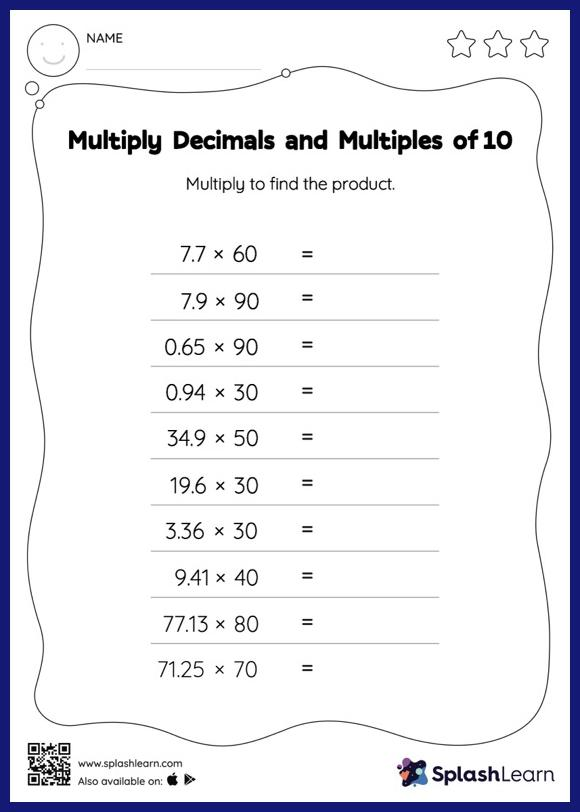# Multiply Decimals and Multiples of 10: Horizontal Multiplication Worksheet

Home > Multiply Decimals and Multiples of 10: Horizontal MultiplicationThis multiply decimals and multiples of 10 worksheet has a set of problems curated to ensure that your child becomes more proficient in multiplication. Students know that when multiplying a decimal by a number ending in zeros, the decimal point is first ignored and then placed in the product as many places from the right as the number of decimal digits in the original number. They practice this idea thoroughly in multiply decimals and multiples of 10 worksheet. In this worksheet, students practice solving problems written in the horizontal format. How numbers are laid out in a problem affects the method a student employs to solve it. Therefore to develop actual fluency and mastery of multiple strategies, students must practice different formats.